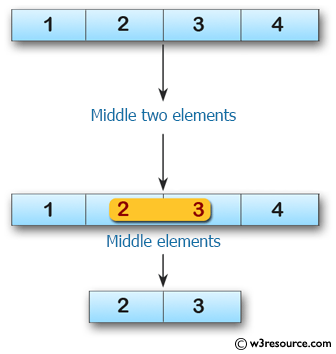﻿ Swift Array Programming Exercise: Create an array of length 2 containing the middle two elements from a given array of integers and even length 2 or more - w3resource# Swift Array Programming Exercises: Create an array of length 2 containing the middle two elements from a given array of integers and even length 2 or more

## Swift Array Programming: Exercise-17 with Solution

Write a Swift program to create an array of length 2 containing the middle two elements from a given array of integers and even length 2 or more.

Pictorial Presentation:Sample Solution:

Swift Code:

``````func middle_elements(_ a: [Int]) -> [Int] {
var new_array: [Int] = []

if a.count % 2 == 0
{
let second_Index = a.count / 2
let first_Index = second_Index - 1
new_array = [a[first_Index], a[second_Index]]
}
return new_array
}

print(middle_elements([1, 2, 3, 4]))
print(middle_elements([5, 6, 7, 8, 9, 10]))
print(middle_elements([5, 6, 7, 8, 9, 10, 11]))
print(middle_elements([1, 2]))
```
```

Sample Output:

```[2, 3]
[7, 8]
[]
[1, 2]
```

Swift Programming Code Editor:

Improve this sample solution and post your code through Disqus

What is the difficulty level of this exercise?

﻿